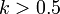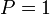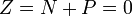# Errata: In Example 9.6, the critical gain is k = 0.5 instead of k = 1

In Example 9.6, the value of the critical gain is listed incorrectly: it should beinstead of. The paragraph after the displayed equation has several changes (highlighted in blue):
We haveand. If, the Nyquist curve encircles the critical pointin the counterclockwise direction when the Nyquist contouris encircled in the clockwise direction. The number of encirclements is thus. Since the loop transfer function has one pole in the right half-plane (), we find thatand the system is thus stable for. If, there is no encirclement and the closed loop will have one pole in the right half-plane.
In addition, in the caption of Figure 9.8, the Nyquist plot is given for gain.